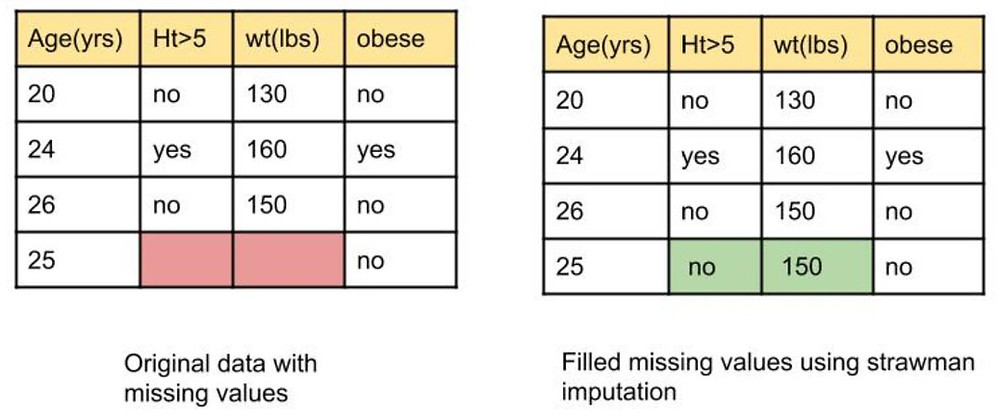Search

# Way to handle missing values-Proximity ImputationMissing data is a pool of problems in the world of data. Data professionals need complete data to analyze and hence are forced to drop the data which may create loss of valuable data and the inferential power. Thus missing data imputation is more reasonable. And the standard ways of filling with median mode have their own challenges and misrepresentation some times there is a need to explore more on other ways of imputation. Let us see in detail about one way of such imputations. It is called Proximity imputation.

In this procedure the data is imputed using Strawman imputation where missing values for continuous variables are replaced using the median of non-missing values, and for missing categorical variables, the most frequently occurring non-missing value is used. A random forest is fit using this data.

Using the resulting forest, proximities are calculated for each pair of observations. If two cases occupy the same terminal node through one tree, their proximity is increased by one. At the end of the run of all trees, the proximities are normalized by dividing by the total number of trees.

For continuous variables, the proximity weighted average of non-missing data is used. For categorical variables, the largest average proximity over non-missing data is used. The updated data is used to grow a new random forest, and the procedure is iterated.

Let us fill the missing values using proximity imputation in the following example. The data here has 2 missing values and they are filled using the strawman imputation that is mode, median(no,150).Now let us refine this data.In order to refine the filled data , first we need to build a random forest with this data and run all the observations through all decision trees and find the similar observations. If 2 observations end at the same leaf node then they are considered similar. Let us assume that 3rd and 4th observations end at the same node after analysis and they both are similar.

We keep track of the similar samples using a proximity matrix where each observation has each row and each column. Since we found 3rd and 4th are similar observations we fill the intersection of these observations with 1 in the matrix as shown in Matrix A.Matrix A is the representation of proximity after running all observations through decision tree 1 of the random forest. Similarly we will fill matrix until all observations are passed through all decision trees of the forest. Let us assume observations 2,3 and 4 ended up in same leaf node of next decision tree, we will fill the matrix as in matrix B. Now if we run the observations for the next decision tree we will fill proximity matrix as Matrix C where observations 3 and 4 ended at same leaf node. Matrix D shows after all the observations are passed through all the decision trees.Now we have to divide the proximity values by the total number of decision trees. Let us assume there are 10 trees here then the matrix will be a matrix E. Now let us use the sample 4 values to refine the filled values.

Now we should calculate the weighted frequency of our assumed values. We need the frequency of yes and no of height column and the weight of yes and no proximities.

The frequency of yes and no are as follows:

Yes = 1/3 No = 2/3

The weight of yes = proximity of yes/ all proximities of the sample = 0.1(blue colored cell in matrix E)/(0.1+0.1+0.8) = 0.1

The weight of no = 0.1+0.8 (red colored cells in matrix E)/0.1+0.1+0.8 = 0.9

The weighted frequency of yes = frequency of yes * the weight for yes = ⅓ * 0.1 =0.03

The weighted frequency of no = ⅔*0.9 = 0.6

The weighted frequency of no is greater than yes, so no would be the good option to fill the missing value of observation 4 height column.

Similarly we need to calculate the weighted frequency for the weight column to refine the filling. To calculate this we need to multiply the observation and weights of the values for each observation as follows.

Observation 1 = 130 * 0.1(first red cell in matrix E)/(0.1+0.1+0.8)= 130 * 0.1 =13

Observation 2 = 160 * 0.1(blue cell in matrix E)/(0.1+0.1+0.8)=160*0.1 = 16

Observation 3 = 150 * 0.8(second red cell matrix E)/0.1+0.1+0.8 = 150 *0.8 = 120

Sum of all above is 149 so the revised value to fill the weight of observation 4 is 149.

To summarize we filled the missing values and revised them using random forests and this process is continued 6-7 times until the values do not change after recalculation.

Conclusion:

Proximities are used in replacing missing data, locating outliers, and finding low-dimensional views of the data.

Bibliography

NCBI, editor. “Random forests missing data algorithms.” https://www.ncbi.nlm.nih.gov/pmc/articles/PMC5796790/.